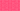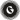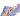PricingResources

# How to calculate WACC (weighted average cost of capital)Written by

Many companies use borrowed funds to run their business, so formulas for calculating the cost of capital are an important element of any assessment of a company’s potential profitability. Used by analysts and investors to evaluate whether a company is worth investing in, WACC is one such formula. But what is WACC? Learn more about weighted average cost of capital and find out how to calculate WACC for yourself.

## What is WACC?

Weighted average cost of capital (WACC) is a calculation of a business’s blended cost of capital. In this calculation, each type of capital is proportionately weighted by its percentage of the total amount of capital, before being added together. When you calculate WACC, you need to include all sources of capital, including bonds, long-term debt, common stock, and so on.

WACC is often used as a hurdle rate (minimum rate of return expected from a project or investment) to evaluate investment opportunities, which can make it a great indicator of whether an investment is worth pursuing or not. In addition, WACC may be used as the discount rate when calculating the Net Present Value (NPV) of a business.

## How to calculate weighted average cost of capital

The standard WACC formula may look a little complicated, but once you’ve got all the information you need, learning how to calculate WACC isn’t too much of a challenge. Here’s the WACC formula:

WACC = (E/V x Re) + ((D/V x Rd) x (1-T))

Where:

• E = Market value of the business’s equity

• V = Total value of capital (equity + debt)

• Re = Cost of equity

• D = Market value of the business’s debt

• Rd = Cost of debt

• T = Tax rate

Essentially, you need to multiply the cost of each capital component with its proportional rate. These results are then multiplied by your business’s corporate tax rate, providing you with a figure for the weighted average cost of capital.

## Calculating cost of equity

When using the WACC formula, calculating cost of equity (Re) is one of the main areas where you could slip up. This is because share capital doesn’t have a concrete price, it’s simply issued to investors for whatever they’re willing to pay.

So, to work out how to calculate cost of equity, we need to look at how investors buy and sell stocks. Put simply, shareholders expect a return on their investment, and failing to provide it to them may lead to sales of your shares, decreasing your company’s value. As a result, the cost of equity is the amount your business needs to spend to maintain a satisfactory share price.

## What can you learn from WACC?

WACC can be an effective way for investors and analysts to determine whether or not to invest in a company. Because WACC provides insight into the average cost of borrowing, a higher weighted average percentage may indicate that a company’s cost of financing is greater. This means that the business will have less cash to pay off additional debt or distribute to shareholders, meaning that it’s less likely to produce value, and may not be a good investment.

## What are the limitations of the WACC formula?

However, there are some disadvantages associated with using WACC to evaluate projects. Most significantly, learning how to calculate WACC appears much simpler than it really is. Some elements, such as cost of equity, aren’t consistent, and different businesses may report them in different ways. This doesn’t give the WACC formula a particularly high level of comparability, and while it’s a useful tool, it shouldn’t be the only metric you use when determining whether to invest in a business.

## We can help

GoCardless helps you automate payment collection, cutting down on the amount of admin your team needs to deal with when chasing invoices. Find out how GoCardless can help you with ad hoc payments or recurring payments.Contact sales

Sales

Support